Courses

# Test: Data Tabulation & Caselets - 2

## 15 Questions MCQ Test Logical Reasoning (LR) and Data Interpretation (DI) | Test: Data Tabulation & Caselets - 2

Description
This mock test of Test: Data Tabulation & Caselets - 2 for CAT helps you for every CAT entrance exam. This contains 15 Multiple Choice Questions for CAT Test: Data Tabulation & Caselets - 2 (mcq) to study with solutions a complete question bank. The solved questions answers in this Test: Data Tabulation & Caselets - 2 quiz give you a good mix of easy questions and tough questions. CAT students definitely take this Test: Data Tabulation & Caselets - 2 exercise for a better result in the exam. You can find other Test: Data Tabulation & Caselets - 2 extra questions, long questions & short questions for CAT on EduRev as well by searching above.
QUESTION: 1

### Directions for Questions: Answer the questions on the basis of the information given below.  The total electricity production of five thermal power plants in India in year 2009-10 is given in the table below. Capacity utilization for any power plant is the percentage of maximum capacity, of that power plant, which is used for power production. Maximum capacity (100%) = Capacity utilization (In %) + Unutilized production (In %) (2015)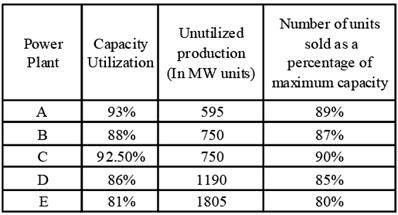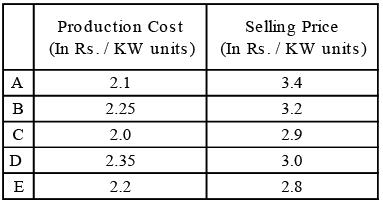Total cost of production = Units Produced (in KW) × Production Cost (in Rs. / KW units) Total Revenue = Units Sold (in KW) × Selling price (in Rs. / KW units) Profitability = (Revenue - Cost / Cost) × 100 Q. Which of the following represents the decreasing order of units sold by the given 5 power plants?

Solution: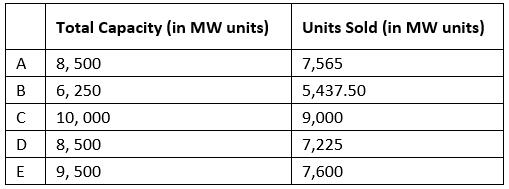According to above table, The correct order is C > E > A > D > B

QUESTION: 2

### Directions for Questions: Answer the questions on the basis of the information given below.  The total electricity production of five thermal power plants in India in year 2009-10 is given in the table below. Capacity utilization for any power plant is the percentage of maximum capacity, of that power plant, which is used for power production. Maximum capacity (100%) = Capacity utilization (In %) + Unutilized production (In %) (2015)Total cost of production = Units Produced (in KW) × Production Cost (in Rs. / KW units) Total Revenue = Units Sold (in KW) × Selling price (in Rs. / KW units) Profitability = (Revenue - Cost / Cost) × 100 Q. Which of the following statements is true?

Solution:Only statement 'b' is true.

QUESTION: 3

### Directions for Questions: Answer the questions on the basis of the information given below.  The total electricity production of five thermal power plants in India in year 2009-10 is given in the table below. Capacity utilization for any power plant is the percentage of maximum capacity, of that power plant, which is used for power production. Maximum capacity (100%) = Capacity utilization (In %) + Unutilized production (In %) (2015)Total cost of production = Units Produced (in KW) × Production Cost (in Rs. / KW units) Total Revenue = Units Sold (in KW) × Selling price (in Rs. / KW units) Profitability = (Revenue - Cost / Cost) × 100 Q. In the given year, if capacity of power plant B had 12.5% of the total power capacity of India, and thermal power capacity of India is 95% of its total power capacity. The total capacity of these 5 thermal power plants was what percentage of the total thermal power capacity of India?

Solution: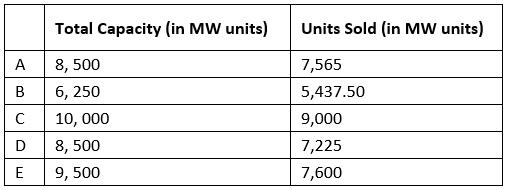∵ Total capacity of power plant B of India = 6250.
∴ Total capacity of India = 6,250 × 100/12.5
= 50,000 MW units
∴ Thermal capacity of India = 95% of total capacity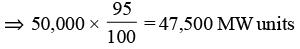Total capacity of these five power plants = (8500 + 6250 + 10,000 + 8500 + 9500) = 42,750 MW units
300 + 3400 = 3700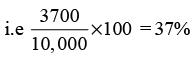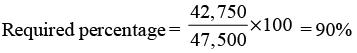QUESTION: 4

Directions for Questions: Answer the questions on the basis of the information given below.
The total electricity production of five thermal power plants in India in year 2009-10 is given in the table below. Capacity utilization for any power plant is the percentage of maximum capacity, of that power plant, which is used for power production.
Maximum capacity (100%) = Capacity utilization (In %) + Unutilized production (In %)

(2015)Total cost of production = Units Produced (in KW) × Production Cost (in Rs. / KW units)
Total Revenue = Units Sold (in KW) × Selling price (in Rs. / KW units)
Profitability = (Revenue - Cost / Cost) × 100

Q. Which power plant had the third highest profitability?

Solution: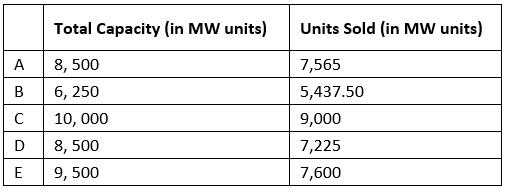Profitability can be compared by comparing the ratio of total revenue to total cost.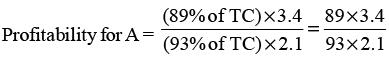= 1.549.
(where TC is the total capacity of that power plant.)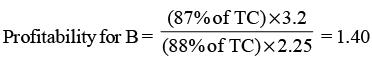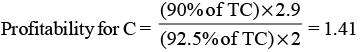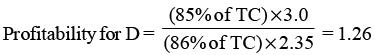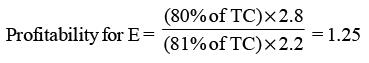So, B has the third highest profitability.

QUESTION: 5

Directions for Questions: Answer the questions on the basis of the information given below.
The table given below shows the data related to a few key financial indicators for fourteen European countries in the FY 2011-12.

(2013)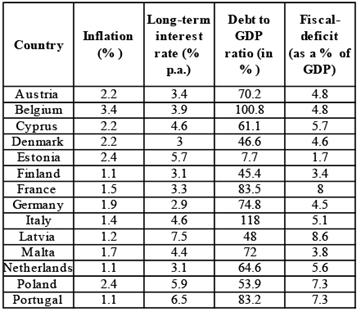Q. If the Fiscal-deficit of France was x Euros, which was 50% more than that of Belgium, then what was the Debt (in Euros) of Belgium in FY 2011-12?

Solution:

Let the GDP of Belgium by E Euros.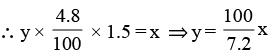Hence, the debt (in Euros) of Belgium) in FY 2011–12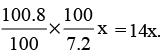QUESTION: 6

Directions for Questions: Answer the questions on the basis of the information given below.
The table given below shows the data related to a few key financial indicators for fourteen European countries in the FY 2011-12.

(2013)Q. The countries with the Long-term interest rate less than 4% per annum, Debt to GDP ratio less than 60% and Fiscal-deficit not more than 4.6% were given a AAA rating. The number of countries rated AAA among the fourteen in FY 2011-12 was

Solution:

According to given data Two countries, viz. Denmark and Finalnd, were rated AAA among the fourteen in FY 2011–12.

QUESTION: 7

Directions for Questions: Answer the questions on the basis of the information given below.
The table given below shows the data related to a few key financial indicators for fourteen European countries in the FY 2011-12.

(2013)Q. If the GDP (in Euros) of Finland was 50% more than that of Italy, then by what percent was the Fiscaldeficit (in Euros) of Italy more/less than that of Finland in FY 2011-12?

Solution:

Let the GDP (in Euros) of Italy = x.
Therefore. the GDP (in Euros) of Finland = 1.5x.
The fiscal–deficit of.
Italy = (x + 5.1 / 100) = 0.051 x
Finland = (1.5x x 3.4 / 100) = 0.034 x 1.5x = 0.051 x.
Hence, the required percentage = 0.

QUESTION: 8

Directions for Questions: Seven companies A, B, C, D, E, F and G are engaged in production of two items I and II. The comparative data about production of these items by the seven companies is given in the following Graph and Table. Study them carefully to answer the questions that follow.

(2011)

PERCENTAGE OF THE TOTAL PRODUCTION PRODUCED BY THE SEVEN COMPANIES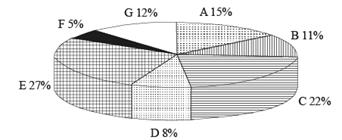Cost of the total production (both items together) by seven companies = ₹ 25 crores
RATIO OF PRODUCTION BETWEEN ITEMS I AND II AND THE PERCENT PROFIT EARNED FOR THE TWO ITEMS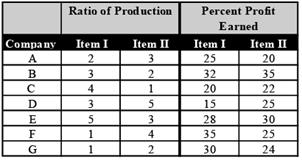Q. Cost of production of item I by company F is what percent of the cost of production of item II by company D?

Solution:

According to the question, cost of total production by all the seven companies = ₹ 25 crores.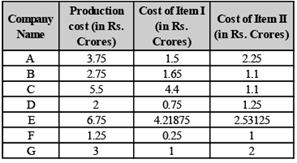Required % age = 0.25 / 1.25 x 100 = 20%

QUESTION: 9

Directions for Questions: Seven companies A, B, C, D, E, F and G are engaged in production of two items I and II. The comparative data about production of these items by the seven companies is given in the following Graph and Table. Study them carefully to answer the questions that follow.

(2011)

PERCENTAGE OF THE TOTAL PRODUCTION PRODUCED BY THE SEVEN COMPANIESCost of the total production (both items together) by seven companies = ₹ 25 crores
RATIO OF PRODUCTION BETWEEN ITEMS I AND II AND THE PERCENT PROFIT EARNED FOR THE TWO ITEMSQ. What is the total profit earned by company G for items I and II together?

Solution:

According to the question, cost of total production by all the seven companies = ₹ 25 crores.Total Profit earned by G for both items I and II = 30% of 1 crore  + 24% of 2 crore = 30 lakhs + 48 lakhs = 78 lakhs.

QUESTION: 10

Directions for Questions: Seven companies A, B, C, D, E, F and G are engaged in production of two items I and II. The comparative data about production of these items by the seven companies is given in the following Graph and Table. Study them carefully to answer the questions that follow.

(2011)

PERCENTAGE OF THE TOTAL PRODUCTION PRODUCED BY THE SEVEN COMPANIESCost of the total production (both items together) by seven companies = ₹ 25 crores
RATIO OF PRODUCTION BETWEEN ITEMS I AND II AND THE PERCENT PROFIT EARNED FOR THE TWO ITEMSQ. What is the ratio of the cost of production of item I by company A to the cost of production of item I by company D?

Solution:

According to the question, cost of total production by all the seven companies = ₹ 25 crores.Cost of production of I by company A / Cost of production of I by company D
= 1.5 / 0.75 = 2 / 1 = 2 : 1

QUESTION: 11

Directions for Questions: Seven companies A, B, C, D, E, F and G are engaged in production of two items I and II. The comparative data about production of these items by the seven companies is given in the following Graph and Table. Study them carefully to answer the questions that follow.

(2011)

PERCENTAGE OF THE TOTAL PRODUCTION PRODUCED BY THE SEVEN COMPANIESCost of the total production (both items together) by seven companies = ₹ 25 crores
RATIO OF PRODUCTION BETWEEN ITEMS I AND II AND THE PERCENT PROFIT EARNED FOR THE TWO ITEMSQ. The cost of production of both items together by company E is equal to the total cost of production of both items together by which of the two companies?

Solution:

According to the question, cost of total production by all the seven companies = ₹ 25 crores.Cost of production of both items together by company E = 6.75 crores Cost of production of C & D = 7.50 crores; of B & G = 5.75 crores; of A and D = 5.75 crores; of C & F = 6.75 crores.

QUESTION: 12

Directions for Questions: Seven companies A, B, C, D, E, F and G are engaged in production of two items I and II. The comparative data about production of these items by the seven companies is given in the following Graph and Table. Study them carefully to answer the questions that follow.

(2011)

PERCENTAGE OF THE TOTAL PRODUCTION PRODUCED BY THE SEVEN COMPANIESCost of the total production (both items together) by seven companies = ₹ 25 crores
RATIO OF PRODUCTION BETWEEN ITEMS I AND II AND THE PERCENT PROFIT EARNED FOR THE TWO ITEMSQ. What is the total of the cost of production of item I by company A and the cost of production of item II by company B?

Solution:

According to the question, cost of total production by all the seven companies = ₹ 25 crores.Cost of production of item I by A and item II by B = 1.5 + 1.10 = 2.60 crores.

QUESTION: 13

Directions for Questions: Read the table carefully and answer the questions that follow:-Given below are data points on the India economy from 2005 - 2010:

(2010)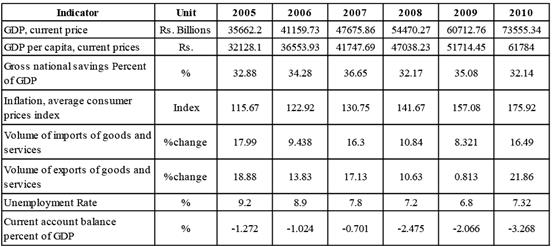*Per capita GDP is arrived by dividing GDP by population.

Q. What is the ratio of the current account balance in 2010 to current account balance 2005?

Solution:

Current account balance in 2010
= – 3.268% of 73555.34
= – 2403.78 (in ₹ billions)
Current account balance in 2005
= – 1.272% of 35662.2
= – 453.62 (in ₹ billions)
∴ Ratio = – 2403.78 / - 453.62 = 5.36 (approx.)

QUESTION: 14

Directions for Questions: Read the table carefully and answer the questions that follow:-Given below are data points on the India economy from 2005 - 2010:

(2010)*Per capita GDP is arrived by dividing GDP by population.

Q. Read the statements given below:
1. Exports were more than imports in 2006
2. Imports were more than exports in 2009
3. Export increased at faster rate than imports during period 2005 to 2010
Which of the statements is necessarily true?

Solution:
QUESTION: 15

Directions for Questions: Read the table carefully and answer the questions that follow:-Given below are data points on the India economy from 2005 - 2010:

(2010)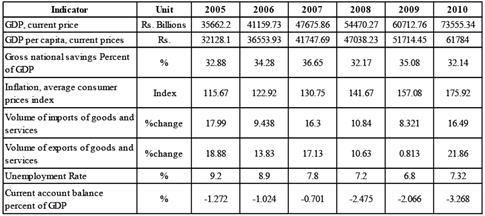*Per capita GDP is arrived by dividing GDP by population.

Q. What was the approximate number of unemployed persons in 2006

Solution:

The number of unemployed persons
= unemployment rate × population
= Unemployment rate × GDP / GDP percapita
= 8.9 / 100 x 41159.73 / 36553.93
= 0.1002 Billion ≈ 100 million.